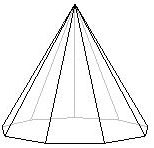### Octagonal Pyramid Volume Calculation

Calculator to find the volume of the octagon pyramid. Please enter the side length (s) and pyramid height (h) to find the volume.
Formula for the volume of an octagonal pyramid = (ba*h) / 3
where ba is the base area of the pyramid and h is the vertical height from the center of the base to the apex.
and formula for the base area (ba) = 2 x s2(1 + √2)
Where s is the side length.Please enter the Side Length (s) = m Please enter thePyramid Height (h) = m Volume (v) = m³
The octagonal pyramid has total nine faces where eight are triangular faces and one face is a regular octagon. The octagonal pyramid also has 23 edges and nine vertices.
Any pyramid having regular polygonal base of n sides will have n+1 faces and vertexes.